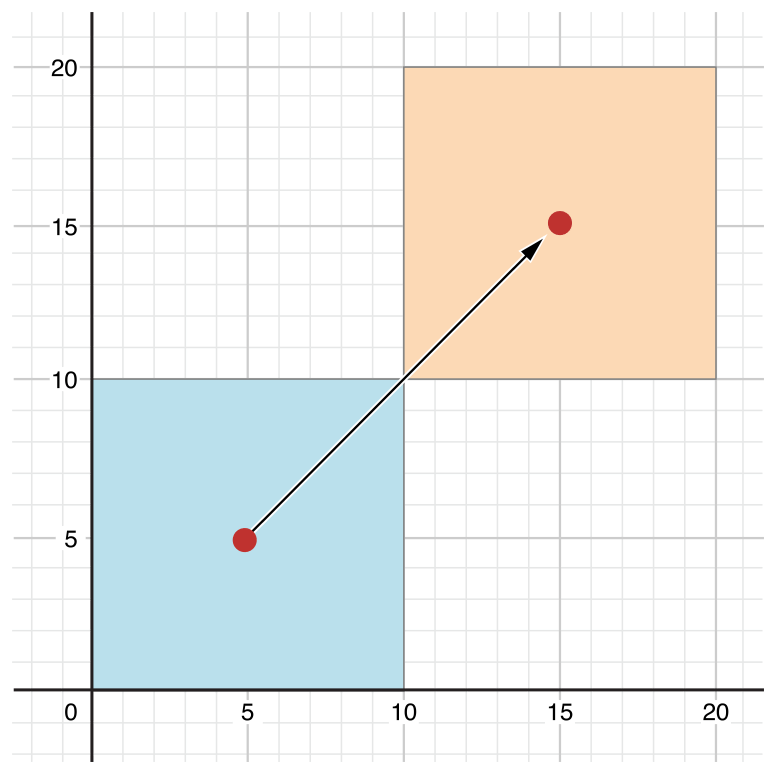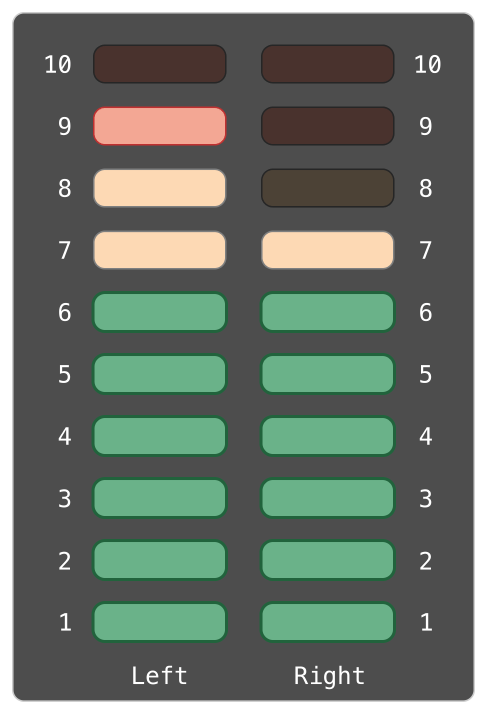# Swift学习之路-属性

## 存储属性

``````struct FixedLengthRange {
var firstValue: Int
let length: Int
}
var rangeOfThreeItems = FixedLengthRange(firstValue: 0, length: 3)
// the range represents integer values 0, 1, and 2
rangeOfThreeItems.firstValue = 6
// the range now represents integer values 6, 7, and 8``````

FixedLengthRange 的实例有一个名为 firstValue 的变量存储属性和一个名为 length 的常量存储属性。在上面的例子中，当新的值域创建时 length 已经被创建并且不能再修改，因为这是一个常量属性。

### 常量结构体实例的存储属性

``````let rangeOfFourItems = FixedLengthRange(firstValue: 0, length: 4)
// this range represents integer values 0, 1, 2, and 3
rangeOfFourItems.firstValue = 6
// this will report an error, even though firstValue is a variable property``````

### 延迟存储属性

``````class DataImporter {

//DataImporter is a class to import data from an external file.
//The class is assumed to take a non-trivial amount of time to initialize.

var fileName = "data.txt"
// the DataImporter class would provide data importing functionality here
}

class DataManager {
lazy var importer = DataImporter()
var data = [String]()
// the DataManager class would provide data management functionality here
}

let manager = DataManager()
manager.data.append("Some data")
manager.data.append("Some more data")
// the DataImporter instance for the importer property has not yet been created``````

DataManager 类的功能之一是从文件导入数据。此功能由 DataImporter 类提供，它假定为需要一定时间来进行初始化。这大概是因为 DataImporter 实例在进行初始化的时候需要打开文件并读取其内容到内存中。

DataManager 实例并不要从文件导入数据就可以管理其数据的情况是有可能发生的，所以当 DataManager 本身创建的时候没有必要去再创建一个新的 DataImporter 实例。反之，在 DataImporter 第一次被使用的时候再创建它才更有意义。

``````print(manager.importer.fileName)
// the DataImporter instance for the importer property has now been created
// prints "data.txt"``````

### 存储属性与实例变量

Swift 把这些概念都统一到了属性声明里。Swift 属性没有与之相对应的实例变量，并且属性的后备存储不能被直接访问。这避免了不同环境中对值的访问的混淆并且将属性的声明简化为一条单一的、限定的语句。所有关于属性的信息——包括它的名字，类型和内存管理特征——都作为类的定义放在了同一个地方。

## 计算属性

``````struct Point {
var x = 0.0, y = 0.0
}
struct Size {
var width = 0.0, height = 0.0
}
struct Rect {
var origin = Point()
var size = Size()
var center: Point {
get {
let centerX = origin.x + (size.width / 2)
let centerY = origin.y + (size.height / 2)
return Point(x: centerX, y: centerY)
}
set(newCenter) {
origin.x = newCenter.x - (size.width / 2)
origin.y = newCenter.y - (size.height / 2)
}
}
}
var square = Rect(origin: Point(x: 0.0, y: 0.0),
size: Size(width: 10.0, height: 10.0))
let initialSquareCenter = square.center
square.center = Point(x: 15.0, y: 15.0)
print("square.origin is now at (\(square.origin.x), \(square.origin.y))")
// prints "square.origin is now at (10.0, 10.0)"``````

• Point 封装了一个 (x,y) 坐标;
• Size 封装了一个 width 和 height ;
• Rect 封装了一个长方形包括原点和大小。

Rect 结构体还提供了一个名为 center 的计算属性。 Rect 的当前中心位置由它的 origin 和 size 决定，所以你不需要把中心点作为一个明确的 Point 值来存储。相反， Rect 为名为 center 的计算变量定义了一个定制的读取器（ getter ）和设置器（ setter ），来允许你使用该长方形的 center ，就好像它是一个真正的存储属性一样。

square 变量的 center 属性通过点操作符（ square. center ）来访问，通过调用 center 的读取器，来得到当前的属性值。而读取器实际上是计算并返回一个新的 Point 来表示这个方形区域的中心，而不是返回一个已存在的值。如上边你看到的，getter正确返回了一个值为（ 5，5 ）的中心点。### 简写设置器（setter）声明

``````struct AlternativeRect {
var origin = Point()
var size = Size()
var center: Point {
get {
let centerX = origin.x + (size.width / 2)
let centerY = origin.y + (size.height / 2)
return Point(x: centerX, y: centerY)
}
set {
origin.x = newValue.x - (size.width / 2)
origin.y = newValue.y - (size.height / 2)
}
}
}``````

### 缩写读取器（getter）声明

``````struct CompactRect {
var origin = Point()
var size = Size()
var center: Point {
get {
Point(x: origin.x + (size.width / 2),
y: origin.y + (size.height / 2))
}
set {
origin.x = newValue.x - (size.width / 2)
origin.y = newValue.y - (size.height / 2)
}
}
}``````

### 只读计算属性

``````struct Cuboid {
var width = 0.0, height = 0.0, depth = 0.0
var volume: Double {
return width * height * depth
}
}
let fourByFiveByTwo = Cuboid(width: 4.0, height: 5.0, depth: 2.0)
print("the volume of fourByFiveByTwo is \(fourByFiveByTwo.volume)")
// prints "the volume of fourByFiveByTwo is 40.0"``````

## 属性观察者

• 你定义的存储属性；
• 你继承的存储属性；
• 你继承的计算属性。

• willSet 会在该值被存储之前被调用。
• didSet 会在一个新值被存储后被调用。

``````class StepCounter {
var totalSteps: Int = 0 {
willSet(newTotalSteps) {
print("About to set totalSteps to \(newTotalSteps)")
}
didSet {
if totalSteps > oldValue  {
}
}
}
}
let stepCounter = StepCounter()
stepCounter.totalSteps = 200
// About to set totalSteps to 200
stepCounter.totalSteps = 360
// About to set totalSteps to 360
stepCounter.totalSteps = 896
// About to set totalSteps to 896

StepCounter 类声明了一个 Int 类型的 totalSteps 属性。这是一个包含了 willSet 和 didSet 观察者的储存属性。

totalSteps 的 willSet 和 didSet 观察者会在每次属性被赋新值的时候调用。就算新值与当前值完全相同也会如此。

didSet 观察者在 totalSteps 的值更新后调用。它用旧值对比 totalSteps 的新值。如果总步数增加了，就打印一条信息来表示接收了多少新的步数。 didSet 观察者不会提供自定义的形式参数名给旧值，而是使用 oldValue 这个默认的名字。

## 属性包装

``````@propertyWrapper
struct TwelveOrLess {
private var number = 0
var wrappedValue: Int {
get { return number }
set { number = min(newValue, 12) }
}
}``````

Setter 保证了新值小于12，getter 返回保存的值。

``````struct SmallRectangle {
@TwelveOrLess var height: Int
@TwelveOrLess var width: Int
}

var rectangle = SmallRectangle()
print(rectangle.height)
// Prints "0"

rectangle.height = 10
print(rectangle.height)
// Prints "10"

rectangle.height = 24
print(rectangle.height)
// Prints "12"``````

height 和 width 属性从 TwelveOrLess 的定义中得到它们的初始值，它设置 `TwelveOrLess.number`为零。设置数字 10 到 `rectangle.height` 可以成功，因为它是一个小数字。尝试存入 24 ，则实际存的是 12，因为 24 对于 setter 的规则来说太大了。

``````struct SmallRectangle {
private var _height = TwelveOrLess()
private var _width = TwelveOrLess()
var height: Int {
get { return _height.wrappedValue }
set { _height.wrappedValue = newValue }
}
var width: Int {
get { return _width.wrappedValue }
set { _width.wrappedValue = newValue }
}
}``````

_height 和 _width 属性存储了一个属性包装的实例， TwelveOrLess 。 height 和 width 的 getter 和 setter 包装了 wrappedValue 属性的访问。

### 设定包装属性的初始值

``````@propertyWrapper
struct SmallNumber {
private var maximum: Int
private var number: Int

var wrappedValue: Int {
get { return number }
set { number = min(newValue, maximum) }
}

init() {
maximum = 12
number = 0
}
init(wrappedValue: Int) {
maximum = 12
number = min(wrappedValue, maximum)
}
init(wrappedValue: Int, maximum: Int) {
self.maximum = maximum
number = min(wrappedValue, maximum)
}
}``````

SmallNumber 的定义包含了三个初始化器—— `init() ``init(wrappedValue:) `、以及 `init(wrappedValue:maximum:)` ——也就是下面例子中用来设置包装值和最大值的初始化器。更多关于初始化以及初始化器的语法，见初始化

``````struct ZeroRectangle {
@SmallNumber var height: Int
@SmallNumber var width: Int
}

var zeroRectangle = ZeroRectangle()
print(zeroRectangle.height, zeroRectangle.width)
// Prints "0 0"``````

``````struct UnitRectangle {
@SmallNumber var height: Int = 1
@SmallNumber var width: Int = 1
}

var unitRectangle = UnitRectangle()
print(unitRectangle.height, unitRectangle.width)
// Prints "1 1"``````

``````struct NarrowRectangle {
@SmallNumber(wrappedValue: 2, maximum: 5) var height: Int
@SmallNumber(wrappedValue: 3, maximum: 4) var width: Int
}

var narrowRectangle = NarrowRectangle()
print(narrowRectangle.height, narrowRectangle.width)
// Prints "2 3"

narrowRectangle.height = 100
narrowRectangle.width = 100
print(narrowRectangle.height, narrowRectangle.width)
// Prints "5 4"``````

``````struct MixedRectangle {
@SmallNumber var height: Int = 1
@SmallNumber(maximum: 9) var width: Int = 2
}

var mixedRectangle = MixedRectangle()
print(mixedRectangle.height)
// Prints "1"

mixedRectangle.height = 20
print(mixedRectangle.height)
// Prints "12"``````

### 通过属性包装映射值

``````@propertyWrapper
struct SmallNumber {
private var number = 0
var projectedValue = false
var wrappedValue: Int {
get { return number }
set {
if newValue > 12 {
number = 12
projectedValue = true
} else {
number = newValue
projectedValue = false
}
}
}
}
struct SomeStructure {
}
var someStructure = SomeStructure()

// Prints "false"

// Prints "true"``````

``````enum Size {
case small, large
}

struct SizedRectangle {
@SmallNumber var height: Int
@SmallNumber var width: Int

mutating func resize(to size: Size) -> Bool {
switch size {
case .small:
height = 10
width = 20
case .large:
height = 100
width = 100
}
return \$height || \$width
}
}``````

## 类型属性

### 类型属性语法

``````struct SomeStructure {
static var storedTypeProperty = "Some value."
static var computedTypeProperty: Int {
return 1
}
}
enum SomeEnumeration {
static var storedTypeProperty = "Some value."
static var computedTypeProperty: Int {
return 6
}
}
class SomeClass {
static var storedTypeProperty = "Some value."
static var computedTypeProperty: Int {
return 27
}
class var overrideableComputedTypeProperty: Int {
return 107
}
}``````

### 查询和设置类型属性

``````print(SomeStructure.storedTypeProperty)
// prints "Some value."
SomeStructure.storedTypeProperty = "Another value."
print(SomeStructure.storedTypeProperty)
// prints "Another value."
print(SomeEnumeration.computedTypeProperty)
// prints "6"
print(SomeClass.computedTypeProperty)
// prints "27"````````````struct AudioChannel {
static let thresholdLevel = 10
static var maxInputLevelForAllChannels = 0
var currentLevel: Int = 0 {
didSet {
if currentLevel > AudioChannel.thresholdLevel {
// cap the new audio level to the threshold level
currentLevel = AudioChannel.thresholdLevel
}
if currentLevel > AudioChannel.maxInputLevelForAllChannels {
// store this as the new overall maximum input level
AudioChannel.maxInputLevelForAllChannels = currentLevel
}
}
}
}``````

AudioChannel 结构体定义了两个存储类型属性来支持它的功能。第一个， thresholdLevel ，定义了最大限度电平。它是对所有 AudioChannel 实例来说是一个常量值 10 。（如下方描述的那样）如果传入音频信号值大于 10 ，则只会被限定在这个限定值上。

AudioChannel 结构体同样定义了存储实力属性叫做 currentLevel ，它表示声道的当前音频电平标量从 0 到 10 。

currentLevel 属性有一个 didSet 属性观察者来检查每次 currentLevel 设定的值，这个观察者执行两个检查：

• 如果 currentLevel 的新值比允许的限定值要大，属性观察者就限定 currentLevel 为 thresholdLevel ；
• 如果 currentLevel 的新值（任何限定之后）比之前任何 AudioChannel实例接收的都高，属性观察者就在 maxInputLevelForAllChannels 类型属性里储存 currentLevel 的新值。

``````var leftChannel = AudioChannel()
var rightChannel = AudioChannel()``````

``````leftChannel.currentLevel = 7
print(leftChannel.currentLevel)
// prints "7"
print(AudioChannel.maxInputLevelForAllChannels)
// prints "7"``````

``````rightChannel.currentLevel = 11
print(rightChannel.currentLevel)
// prints "10"
print(AudioChannel.maxInputLevelForAllChannels)
// prints "10"``````